# Comp2Exchange

Model number
0245

Models two compartments with a single substance passively exchanging between the two compartments.

## Description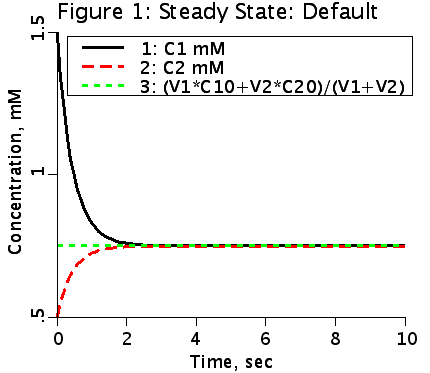This is a model of two compartments, one with volume V1 and initial concentration C10, and the other with volume V2 and concentration C20. The two compartments exchange material at a rate PS. PS is the product of the permeability multiplying the surface area. The exchange is passive and equal in both directions in this model.

## Equations

#### Ordinary Differential Equations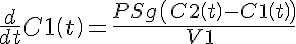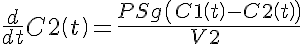#### Initial Conditions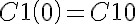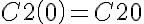#### Analytic Solutions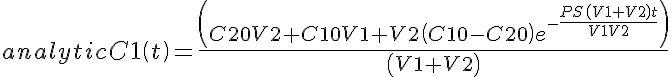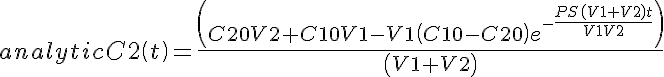The equations for this model may be viewed by running the JSim model applet and clicking on the Source tab at the bottom left of JSim's Run Time graphical user interface. The equations are written in JSim's Mathematical Modeling Language (MML). See the Introduction to MML and the MML Reference Manual. Additional documentation for MML can be found by using the search option at the Physiome home page.

References

None.

Key terms
Course
compartment
compartmental
tutorial
exchange
multiple compartments
flux